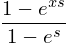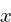expmap

# expmap

### Overview

expmap is an exponential signal mapper with variable slope. It takes in a normalized linear value, and applies an exponential curve to it.

Two version of expmap are provided: a naive implementation that is easy to read and adapt, and a more optimized version that uses parameter caching to reduce division operations and calls to exp.

### Equation

This is all there is to it:Whereis the normalized linear input signal, andis the slope.

Asgets more negative, the exponential curve will be more convex, with more values staying closer to 1. Asgets more positive, the exponential curve will get more concave, with more values staying closer to 0.

### Tangled Files

expmap.c and expmap.h are the tangled files. If SK_EXPMAP_PRIV is defined, it exposes the structs.

<<expmap.c>>=
#include <math.h>
#define SK_EXPMAP_PRIV
#include "expmap.h"
<<static_funcdefs>>
<<funcs>>

<<expmap.h>>=
#ifndef SK_EXPMAP_H
#define SK_EXPMAP_H

#ifndef SKFLT
#define SKFLT float
#endif

<<typedefs>>
<<funcdefs>>

#ifdef SK_EXPMAP_PRIV
<<structs>>
#endif

#endif

### Naive Function (stateless).

The function sk_expmap_stateless will compute this exponential function without any internal state. The code is quite clear and simple, but requires two calls exp, which is an expensive operation.

<<funcdefs>>=
SKFLT sk_expmap_stateless(SKFLT in, SKFLT slope);

<<funcs>>=
SKFLT sk_expmap_stateless(SKFLT in, SKFLT slope)
{
return (1 - exp(in*slope)) / (1 - exp(slope));
}

### Struct

Called sk_expmap.

<<typedefs>>=
typedef struct sk_expmap sk_expmap;

<<structs>>=
struct sk_expmap {
<<sk_expmap>>
};

### Input/Ouput Caching

To avoid computation, some caching of the input/output variables are used.

pin is the cached input value, will be used to avoid computation. At init, it is set to be an (invalid) negative value.

<<sk_expmap>>=
SKFLT pin;

<<init>>=
em->pin = -1;

pout is the cached computed output value. It is used if no computation is needed. By default, it is set to be -1. It is assumed that this value shouldn't actually ever be returned.

<<sk_expmap>>=
SKFLT pout;

<<init>>=
em->pout = -1;

### Scaling Constant

scale is the constant 1/(1 - exp(slope). A scaler used to shave off an expensive exp operation and div operation.

<<sk_expmap>>=
SKFLT scale;

The scaling value can be computed with the static function compute_scale, given an input slope.

<<static_funcdefs>>=
static SKFLT compute_scale(SKFLT slope);

<<funcs>>=
static SKFLT compute_scale(SKFLT slope)
{
return 1 / (1 - exp(slope));
}

After the slope is initialized, the scale is initialized to be the slope.

<<init_scale>>=
em->scale = compute_scale(em->slope);

### Initialization

Initialization is done with sk_expmap_init.

<<funcdefs>>=
void sk_expmap_init(sk_expmap *em);

<<funcs>>=
void sk_expmap_init(sk_expmap *em)
{
<<init>>
}

### Changing Slope

The slope of expmap can be changed with sk_expmap_slope.

<<funcdefs>>=
void sk_expmap_slope(sk_expmap *em, SKFLT slope);

<<funcs>>=
void sk_expmap_slope(sk_expmap *em, SKFLT slope)
{
em->slope = slope;
}

The slope parameter uses caching to avoid re-computation.

<<sk_expmap>>=
SKFLT slope;
SKFLT pslope;

The slope is set to be 1 to begin.

<<init>>=
sk_expmap_slope(em, 1);
em->pslope = 1;
<<init_scale>>

### Compute

A single sample of audio is computed with sk_expmap_tick.

<<funcdefs>>=
SKFLT sk_expmap_tick(sk_expmap *em, SKFLT in);

<<funcs>>=
SKFLT sk_expmap_tick(sk_expmap *em, SKFLT in)
{
SKFLT out = 0;
<<update_scale_value>>
<<compute>>
return out;
}

Before a sample is computed, the slope parameter is checked for updates. If it is updated, the scale needs to be re-computed.

<<update_scale_value>>=
if (em->slope != em->pslope) {
em->pslope = em->slope;
em->scale = compute_scale(em->slope);
}

Computation is done only if the input value in is different from the cached input value pin. If it is, it updates the cached output value pout.

the output variable out is set to be the cached value pout.

<<compute>>=
if (in != em->pin) {
em->pin = in;
em->pout = (1 - exp(in * em->slope)) * em->scale;
}
out = em->pout;